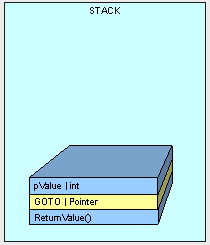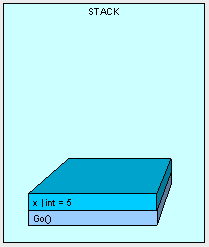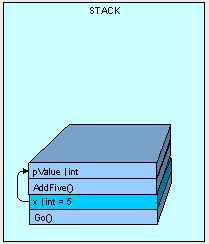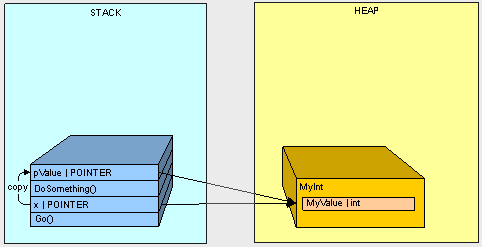﻿ .NET中栈和堆的比较(2) - .Net知识问答 - 郑州网建

# .NET中栈和堆的比较(2)

* 参数，大问题

1.方法执行时，首先在栈上为对象实例中的方法分配空间，然后将方法拷贝到栈上（此时的栈被称为帧），但是该空间中只存放了执行方法的指令，并没有方法内的数据项。

3.方法参数的分配和拷贝是需要空间的，这一点是我们需要进一步注意。

{
int result;
result = pValue + 5;
return result;
}* 值类型传递

class Class1
{

public void Go()
{

int x = 5;

Console.WriteLine(x.ToString());
}

{

pValue += 5;

return pValue;
}
}public struct MyStruct

{

long a, b, c, d, e, f, g, h, i, j, k, l, m;

}

public void Go()

{

MyStruct x = new MyStruct();

DoSomething(x);

}

public void DoSomething(MyStruct pValue)

{

// DO SOMETHING HERE....

}public void Go()

{

MyStruct x = new MyStruct();

DoSomething(ref x);

}

public struct MyStruct

{

long a, b, c, d, e, f, g, h, i, j, k, l, m;

}

public void DoSomething(ref MyStruct pValue)

{

// DO SOMETHING HERE....

}public void Go()

{

MyStruct x = new MyStruct();

x.a = 5;

DoSomething(ref x);

Console.WriteLine(x.a.ToString());

}

public void DoSomething(ref MyStruct pValue)

{

pValue.a = 12345;

}

* 引用类型传递

public class MyInt

{

public int MyValue;

}

public void Go()

{

MyInt x = new MyInt();

}public void Go()

{

MyInt x = new MyInt();

x.MyValue = 2;

DoSomething(x);

Console.WriteLine(x.MyValue.ToString());

}

public void DoSomething(MyInt pValue)

{

pValue.MyValue = 12345;

}1.方法Go()入栈
2.Go()方法中的变量x入栈
3.方法DoSomething()入栈
4.参数pValue入栈
5.ｘ的值(MyInt对象的在栈中的指针地址)被拷贝到pValue中

public class Thing

{

}

public class Animal:Thing

{

public int Weight;

}

public class Vegetable:Thing

{

public int Length;

}

public void Go()

{

Thing x = new Animal();

Switcharoo(ref x);

Console.WriteLine(

"x is Animal    :   "

+ (x is Animal).ToString());

Console.WriteLine(

"x is Vegetable :   "

+ (x is Vegetable).ToString());

}

public void Switcharoo(ref Thing pValue)

{

pValue = new Vegetable();

}

x is Animal    :   False
x is Vegetable :   True1.Go()方法入栈
2.x指针入栈
3.Animal对象实例化到堆中
4.Switcharoo()方法入栈
5.pValue入栈且指向ｘ6.Vegetable对象实例化到堆中
7.x的值通过被指向Vegetable对象地址的pValue值所改变。